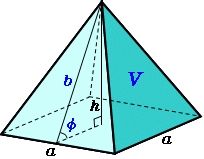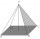# Tetrahedral pyramid

Calculate the volume and surface of the regular tetrahedral pyramid if content area of the base is 20 cm2 and deviation angle of the side edges from the plane of the base is 60 degrees.

Correct result:

V =  36.51 cm3
S =  72.915 cm2

#### Solution:

$S_{1}=20 \ \text{cm}^2 \ \\ Φ=60 \ ^\circ \ \\ \ \\ S_{1}=a^2 \ \\ a=\sqrt{ S_{1} }=\sqrt{ 20 } \doteq 2 \ \sqrt{ 5 } \ \text{cm} \doteq 4.4721 \ \text{cm} \ \\ \ \\ u=\sqrt{ 2 } \cdot \ a=\sqrt{ 2 } \cdot \ 4.4721 \doteq 2 \ \sqrt{ 10 } \ \text{cm} \doteq 6.3246 \ \text{cm} \ \\ \ \\ h=\dfrac{ u }{ 2 } \cdot \ \tan Φ ^\circ =\dfrac{ u }{ 2 } \cdot \ \tan 60^\circ \ =\dfrac{ 6.32455532034 }{ 2 } \cdot \ \tan 60^\circ \ =\dfrac{ 6.32455532034 }{ 2 } \cdot \ 1.732051=5.47723 \ \\ \ \\ V=\dfrac{ 1 }{ 3 } \cdot \ S_{1} \cdot \ h=\dfrac{ 1 }{ 3 } \cdot \ 20 \cdot \ 5.4772=36.51 \ \text{cm}^3$
$b=\sqrt{ h^2 + (a/2)^2 }=\sqrt{ 5.4772^2 + (4.4721/2)^2 } \doteq \sqrt{ 35 } \ \text{cm} \doteq 5.9161 \ \text{cm} \ \\ \ \\ S_{2}=a \cdot \ b/2=4.4721 \cdot \ 5.9161/2 \doteq 5 \ \sqrt{ 7 } \ \text{cm}^2 \doteq 13.2288 \ \text{cm}^2 \ \\ \ \\ S=4 \cdot \ S_{2} + S_{1}=4 \cdot \ 13.2288 + 20=72.915 \ \text{cm}^2$Our examples were largely sent or created by pupils and students themselves. Therefore, we would be pleased if you could send us any errors you found, spelling mistakes, or rephasing the example. Thank you!

Please write to us with your comment on the math problem or ask something. Thank you for helping each other - students, teachers, parents, and problem authors.Tips to related online calculators
Pythagorean theorem is the base for the right triangle calculator.
Tip: Our volume units converter will help you with the conversion of volume units.

#### You need to know the following knowledge to solve this word math problem:

We encourage you to watch this tutorial video on this math problem:

## Next similar math problems:

• Tetrahedral pyramid 8Let’s all side edges of the tetrahedral pyramid ABCDV be equally long and its base let’s be a rectangle. Determine its volume if you know the deviations A=40° B=70° of the planes of adjacent sidewalls and the plane of the base and the height h=16 of the p
• Hexagonal pyramidCalculate the volume and surface area of a regular hexagonal pyramid with a base edge a = 30 m and a side edge b = 50 m.In a regular quadrilateral pyramid, the height is 6.5 cm and the angle between the base and the side wall is 42°. Calculate the surface area and volume of the body. Round calculations to 1 decimal place.A regular quadrilateral pyramid has a volume of 24 dm3 and a base edge a = 4 dm. Calculate: a/height of the pyramid b/sidewall height c/surface of the pyramidA quadrilateral pyramid, which has a rectangular base with dimensions of 24 cm, 13 cm. The height of the pyramid is 18cm. Calculate 1/the area of the base 2/casing area 3/pyramid surface 4/volume of the pyramidThe quadrilateral pyramid has a rectangular base of 24 cm x 3.2dm and a body height of 0.4m. Calculate its volume and surface area.
• The tentCalculate how much cover (without a floor) is used to make a tent that has the shape of a regular square pyramid. The edge of the base is 3 m long and the height of the tent is 2 m.
• The bus stopThe bus stop waiting room has the shape of a regular quadrilateral pyramid 4 m high with a 5 m base edge. Calculate how many m2 roofing is required to cover the three walls of the sheathing, taking into account 40% of the additional coverage.
• Hexagonal pyramidCalculate the surface area of a regular hexagonal pyramid with a base inscribed in a circle with a radius of 8 cm and a height of 20 cm.Calculate the surface of a quadrilateral pyramid, which has a rectangular base with dimensions a = 8 cm, b = 6 cm and height H = 10 cm.
• Rectangular base pyramidCalculate an area of the shell of the pyramid with a rectangular base of 2.8 m and 1.4 m and height 2.5 meters.
• Wall heightCalculate the height of a regular hexagonal pyramid with a base edge of 5 cm and a wall height w = 20 cm.
• Hexagonal pyramidFind the volume of a regular hexagonal pyramid, the base edge of which is 12 cm long and the side edge 20 cm.
• Hexagonal pyramidFind the area of a shell of the regular hexagonal pyramid, if you know that its base edge is 5 cm long and the height of this pyramid is 10 cm.
• Top of the towerThe top of the tower has the shape of a regular hexagonal pyramid. The base edge has a length of 1.2 m, the pyramid height is 1.6 m. How many square meters of sheet metal is needed to cover the top of the tower if 15% extra sheet metal is needed for joint
• Distance of pointsA regular quadrilateral pyramid ABCDV is given, in which edge AB = a = 4 cm and height v = 8 cm. Let S be the center of the CV. Find the distance of points A and S.
• Height of pyramidThe pyramid ABCDV has edge lengths: AB = 4, AV = 7. What is its height?